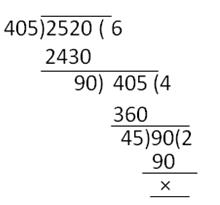Courses

# RS Aggarwal Test: Real Numbers

## 10 Questions MCQ Test Mathematics (Maths) Class 10 | RS Aggarwal Test: Real Numbers

Description
This mock test of RS Aggarwal Test: Real Numbers for Class 10 helps you for every Class 10 entrance exam. This contains 10 Multiple Choice Questions for Class 10 RS Aggarwal Test: Real Numbers (mcq) to study with solutions a complete question bank. The solved questions answers in this RS Aggarwal Test: Real Numbers quiz give you a good mix of easy questions and tough questions. Class 10 students definitely take this RS Aggarwal Test: Real Numbers exercise for a better result in the exam. You can find other RS Aggarwal Test: Real Numbers extra questions, long questions & short questions for Class 10 on EduRev as well by searching above.
QUESTION: 1

### √7 is

Solution:

Assume that √7 be a rational number.
i.e. √7 = p/q, where p and q are co prime.
⇒ 7 = p2/q2
⇒ 7q= p2    ...(1)
⇒ p2 is divisible by 7, i.e. p is divisible by 7
⇒ For any positive integer c, it can be said that p = 7c, p2 = 49c2

Equation (1) can be written as: 7q2 = 49c2
⇒ q2 = 7c2
This gives that q is divisible by 7.

Since p and q have a common factor 7 which is a contradiction to the assumption that they are co-prime.
Therefore, √7 is an irrational number.

QUESTION: 2

### For positive integers a and 3, there exist unique integers q and r such that a = 3q + r, where r must satisfy:

Solution:

Euclid's Division Lemma states that for any two positive integers ‘a’ and ‘b’ there exist two unique whole numbers ‘q’ and ‘r’ such that, a = bq + r, where 0≤ r < b.
Where, a= Dividend, b= Divisor, q= quotient and r = Remainder.

Given: a = 3q+r
⇒ The values 'r’ can take 0 ≤ r < 3.

QUESTION: 3

### For some integer q, every odd integer is of the form

Solution:

We know those odd integers are 1, 3, 5, ...
So, it can be written in the form of 2q + 1, where, q = integer = Z
⇒ q = ..., -1, 0,1,2,3, ...
2q + 1 = ..., -3, -1, 1, 3,5, ...

Alternate Method
Let 'a' be given positive integer. On dividing 'a' by 2, let q be the quotient and r be the remainder.
By Euclid's division algorithm, we have
a = 2q + r, where 0 ≤ r < 2
⇒ a = 2q + r, where r = 0 or r = 1
⇒ a = 2q or 2q + 1
when a = 2q + 1 for some integer q, then clearly a is odd.

QUESTION: 4

Find the greatest number of 5 digits, that will give us remainder of 5, when divided by 8 and 9 respectively.

Solution:

Greatest 5-Digit number = 99999

LCM of 8 and 9,

8 = 2 × 2 × 2

9 = 3 × 3

LCM = 2 × 2 × 2 × 3 × 3 = 72

Now, dividing 99999 by 72, we get

Quotient = 1388

Remainder = 63

So, the greatest 5-digit number divisible by 8 and 9 = 99999 - 63 = 99936

Required number = 99936 + 5 = 99941

QUESTION: 5

The product of two consecutive integers is divisible by

Solution:

As the case is of 2 consecutive integers, one will be odd and the other one even,
⇒ Their product will always be even i.e. it will always be divisible by an even no., i.e. 2, here.

QUESTION: 6

If two positive integers a and b are written as a = x3y2 and b = xy3, where x, y are prime numbers, then LCM(a, b) is

Solution:

Here, a = x3y2 and b = xy3.
⇒ a = x * x * x * y * y and b = xy * y * y
∴ LCM(a, b) = x * y * y = x3 * y3 = x3y3
LCM = x3y3

QUESTION: 7

n2 - 1 is divisible by 8 if n is

Solution:

Option A: If n is integer similarly n²- 1 is not divisible by 8.
Option B: If n is a natural number then it is not possible for n=1,2.
Option C: If n is odd the possible for n is 3,5,7...
Option D: If n is even then it is not possible for n=2.

Thus, the option C is correct.

QUESTION: 8

If two positive integers p and q can be expressed as p = ab2 and q = a3b; where a, b being prime numbers, then LCM (p, q) is equal to

Solution:

As per question, we have,
p = ab2 = a × b × b
q = a3b = a × a × a × b
So, their Least Common Multiple (LCM) = a3 × b2

QUESTION: 9

The least perfect square number which is divisible by 3, 4, 5, 6 and 8 is

Solution:

L.C.M. of 3, 4, 5, 6, 8 = 2 × 2 × 2 × 3 × 5 = 120
Pair of 2, 3 and 5 is not completed.
To make it a perfect square, the number should be multiplied by 2, 3, 5.

Required number = 120 x 2 x 3 x 5 = 3600.

QUESTION: 10

The ratio between the LCM and HCF of 5,15, 20 is:

Solution:

Factors are following:
5 = 5 x 1
15 = 5 x 3
20 = 2 x 2 x 5

LCM = 5 x 3 x 2 x 2 = 60
HCF = 5
Ratio = LCM/HCF = 60/5 = 12/1 = 12:1

Track your progress, build streaks, highlight & save important lessons and more!

### Similar Content### Related tests